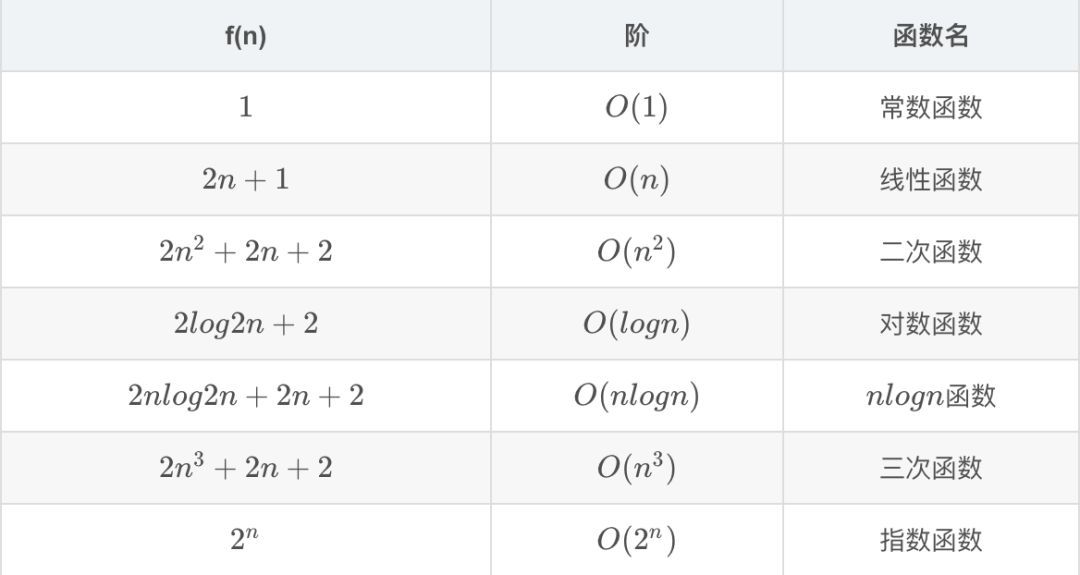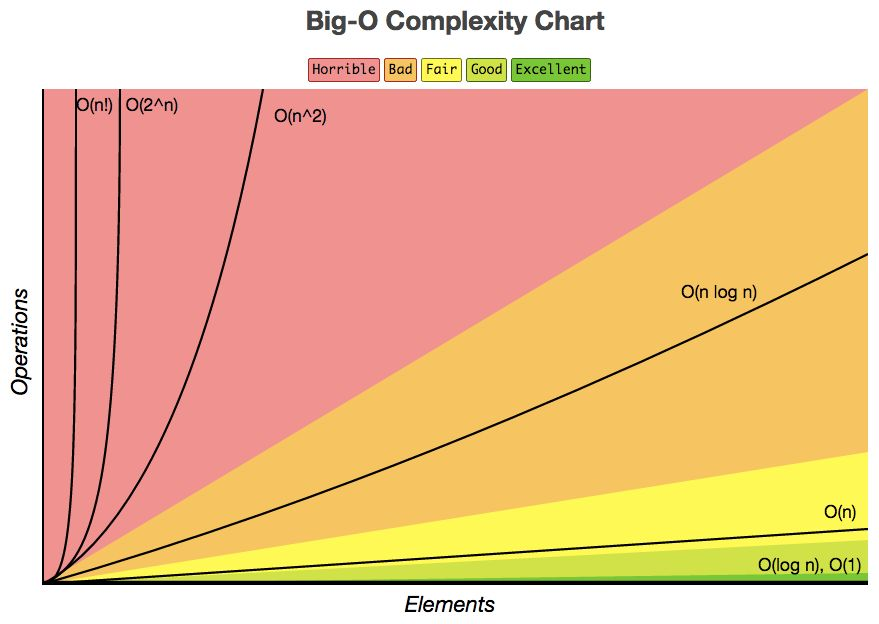# 时间复杂度

## 大 O 表示法

「数量级」函数用来描述当规模 n 增加时，T(n) 函数中增长最快的部分，这个数量级函数我们一般用「大 O」表示，记做 O(f(n))。它提供了计算过程中实际步数的近似值，函数 f(n) 是原始函数 T(n) 中主导部分的简化表示。

## 最好情况、最坏情况和平均情况O(1) < O(logn) < O(n) < O(nlogn) < O(n^2) < O(n^3) < O(2^n)

1. 常数函数

2. 对数函数

) = 3+3n^2 +2n+1 = 3n^2+2n+4，看到指数项，我们自然的发现是 n^2 做主导，当 n 增大时，后面两项可以忽略掉，所以这个代码片段的数量级就是 O(n^2)。

# 后话0%